#### You may also like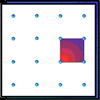### Geoboards

This practical challenge invites you to investigate the different squares you can make on a square geoboard or pegboard.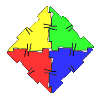### Polydron

This activity investigates how you might make squares and pentominoes from Polydron.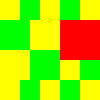### Tiles on a Patio

How many ways can you find of tiling the square patio, using square tiles of different sizes?

# My New Patio

##### Age 7 to 11 Challenge Level:

In the problem Tiles on a Patio , we were looking at tiling a patio. Some of you probably did that and had a good time. If you did not you might like to look back at that challenge and see what you come up with.

But, you know, when you look at gardens or patios you tend to find that they're not lovely rectangles or squares. Someone, quite sensibly, has a bush or small tree growing in it so that there are some tiles missing. Other people have a small pond or a sand pit for smaller brothers and sisters to use. So, what shall we try in this problem?

I thought that we would look at squares, but keep in mind these trees, bushes, sandpits etc. , and so we leave a square tile out.

Let's suppose that we have a space to tile that is $3$ x $3$ and we remove just one tile. It'll look a bit like this:-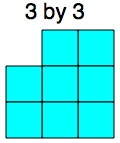Now like in the original challenge you can tile the area using only square tiles. But how about using as few tiles as possible? Perhaps this is the way?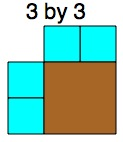There's not a lot of choice there so let's look at a larger area, like this one:-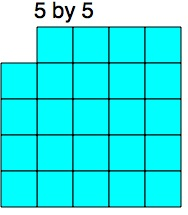Now, after playing around for some time I come to this arrangement, using $1$s, $2$s, and a $3$.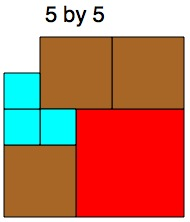That uses a total of seven tiles. Now that seven is the key number that we want for each size square.

The $3$ x $3$ area used five tiles.
The $5$ x $5$ area used seven tiles.

So my challenge ends up with asking you to explore the SMALLEST number of tiles needed to tile a square area when a $1$ x $1$ space is missed out. You do not have to have the one that is missed out in the top left hand corner like I have done. You can have that hole [bush, pond etc. ] wherever you choose.

Well after you have done a few, stop and look at your results and be a 'Numbers Detective' and see what comes up.

If you are doing this with others I suggest you check each other's work to make sure that you have got the smallest number of tiles each time!

You will find probably that the bigger and bigger the area gets, the more excitement that comes!!

So, I went up to $29$ x $29$ for my area, how about you? Of course I have to ask the question:-

I wonder what would happen if ... it were triangles instead?
I wonder what would happen if ... there were two small squares missing?
I wonder what would happen if ... it was a rectangle of a particular area that was not a square?

Good luck!